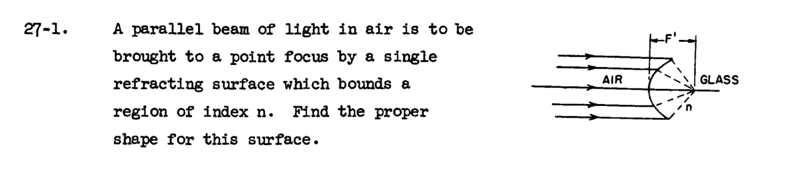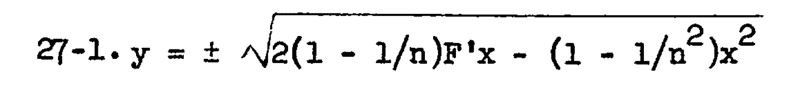# Feynman Exercise 27-1: Shape of glass surface to converge a light beam inside the glass

## Homework Statement[/B]

## Homework Equations

1/s+n/s′=1/f
where
s is distance from source to diffracting surface,
s' is distance from diffracting surface to focus,
f is the focal length,
n is the refractive index.

## The Attempt at a Solution

Since we have parallel beams, we have s = infinity so the equation reduces to
n/s' = 1/F' <=> s' = n*F'
If our coordinate system is placed so that we have origo at F', we get:
s' = sqrt(x^2 + y^2)
inserting and solving for y we get:
sqrt(x^2 + y^2) = n*F'
x^2 + y^2 = (n*F')^2
y = +/- sqrt( (n*F')^2 - x^2 )
But the solution in the book says:What am i doing wrong?

Also, i notice that my solution is a spherical surface with a radius n*F', but the books solution is ellipsical. From optics we know that it should be a spherical surface, so is the book simply wrong?

#### Attachments

Last edited by a moderator:

mjc123
Homework Helper
I don't know the answer, but from the diagram it seems that when y = 0, x = F', and that is not a solution to your equation or the book's.

I don't know the answer, but from the diagram it seems that when y = 0, x = F', and that is not a solution to your equation or the book's.
I don't think that's right. In the problem the origin is not specified. In the books' solution we have a point at x=0, y=0, so the origin must be on the surface of the lens itself.

haruspex
Homework Helper
Gold Member
1/s+n/s′=1/f
Isn't that an approximation?
How about you start from first principles and say that the optical path length is the same for all rays?

••